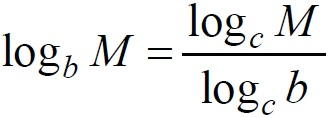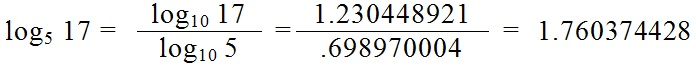# Logarithms: Change of Base

Back to “Basic Algebra” Page

Sometimes a test or an assignment will tell you to solve something ridiculous like this:

17 = 5x

And they want an actual approximate answer!

You might try to solve with this:

log5 17 = x

But unless you’ve spent 150 bucks on a fancy calculator that solves for log5, you won’t get an answer. But you can use a 12-dollar Wal-Mart calculator to solve this, if you know the change of base formula.Remember that the “log” button on your simple calculator is actually log10. So, using the change of base formula for

log5 17 = x

You will getPlugging that answer back into the original equation will give you

17 = 51.760374428 = 17.00000001

which is a very good approximation.

When there is no subscript number after “log” you can assume that it means log10.

Use the flashcards below to practice the change of base formula.

• log2 3 =
log 3 / log 2
• log3 7 =
log 7 / log 3
• log7 11 =
log 11 / log 7
• log19 23 =
log 23 / log 19
• log29 31 =
log 31 / log 29
• log37 41 =
log 41 / log 37